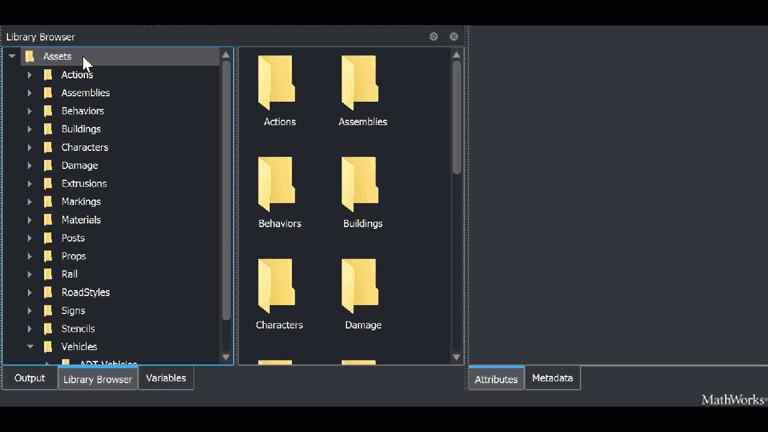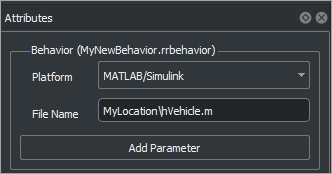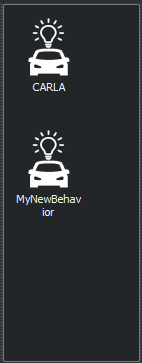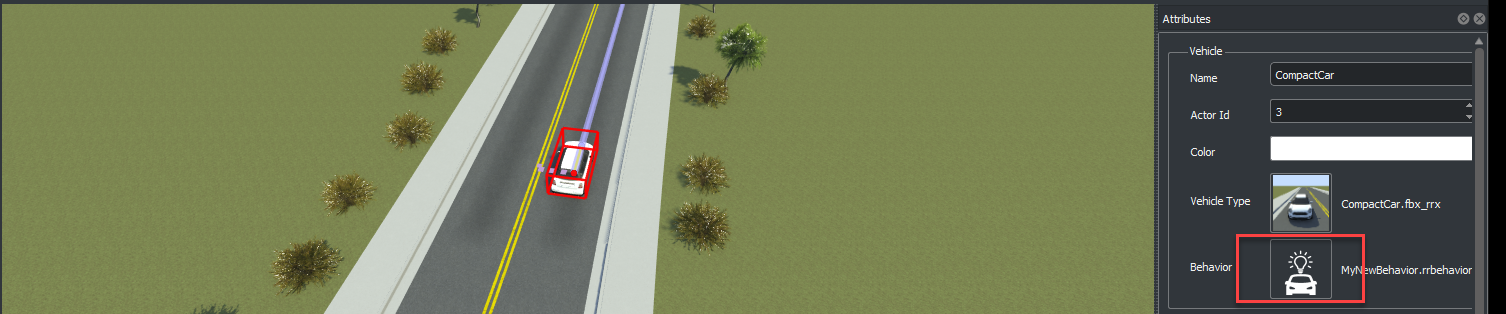## Simulate RoadRunner Scenarios with Actors Modeled in MATLAB

You can use the `ActorSimulation` class for actor modeling and runtime data exchanges with RoadRunner. The sample code examples below show how to program a System object™ file to model scenarios with different kinds of actor behaviors, and then associate these behaviors to your scenario.

To simulate these actor behaviors, you must connect RoadRunner and MATLAB®. This topic provides basic instructions for creating this connection; for more detailed information, see Connect MATLAB and RoadRunner to Control and Analyze Simulations.

These examples assume that:

• You have a RoadRunner Scenario license and the product is installed.

• You have created RoadRunner project folder named `MyRoadRunnerProject`. For more information, see RoadRunner Project and Scene System (RoadRunner).

• You have created and saved a RoadRunner scene named `MyExampleScene` and a scenario named `MyExampleScenario` for your project.

### Build Custom MATLAB System Object Behavior

#### Lane Following Actor Behavior

In this example you create a custom behavior, `hVehicle.m` as a MATLAB System object file. In this behavior, the code reads the initial pose and velocity of an actor and updates them to make the actor follow a lane.

In the custom behavior:

• This code defines the sample time.

``` function st = getSampleTimeImpl(obj) st = createSampleTime( ... obj, 'Type', 'Discrete', 'SampleTime', 0.02); end```
• The code in `setupImpl` is called only once, at the simulation start.

• This code finds the scenario simulation object, which is the scenario with the actors in it.

``` obj.mScenarioSimulationHdl = ... Simulink.ScenarioSimulation.find( ... 'ScenarioSimulation', 'SystemObject', obj);```
• This code uses `Simulink.ScenarioSimulation.find` function and finds the actor object and reflects the actor to which the behavior is attached.

``` obj.mActorSimulationHdl = Simulink.ScenarioSimulation.find( ... 'ActorSimulation', 'SystemObject', obj);```
• The code in `stepImpl` is executed during each time step of a scenario simulation.

• This code calculates the simulation time elapsed since the last step and sets the last simulation time to the current time.

``` currentTime = obj.getCurrentTime; elapsedTime = currentTime - obj.mLastTime; obj.mLastTime = currentTime;```
• This code gets the current pose and velocity of the actor in the scenario.

``` velocity = obj.mActor.velocity; pose = obj.mActor.pose;```
• This code updates the pose.

``` pose(1,4) = pose(1,4) + velocity(1) * elapsedTime; % x pose(2,4) = pose(2,4) + velocity(2) * elapsedTime; % y pose(3,4) = pose(3,4) + velocity(3) * elapsedTime; % z obj.mActor.pose = pose;```
• This code updates RoadRunner actor in the scenario with the new pose values.

`obj.mActorSimulationHdl.setAttribute('Pose', pose);`

#### Path Following Actor Group Behavior

In this example you create a custom behavior for an actor group comprising of a truck and a trailer. The custom behavior `hTruckWithTrailer.m` is written as a MATLAB System object file. In this behavior, the code reads the pose and velocity of the truck (parent) and updates the trailer (child) in such a way that the child remains at a constant distance behind the parent throughout the simulation.

• The code in `stepImpl` is executed during each time step of a scenario simulation.

In the above program, the code in `stepImpl` executes the following logic.

• This code sets a course for the truck (parent) by reading the target path structure.

``` path_action = obj.mActorSimulationHdl.getAction('PathAction'); if(~isempty(path_action)) obj.mActor.path = path_action.PathTarget.Path; obj.mActor.numPts = path_action.PathTarget.NumPoints; obj.mActor.currPt = 1; end```
• This code reads the target speed value.

``` speedChg_action = obj.mActorSimulationHdl.getAction('SpeedAction'); if(~isempty(speedChg_action)) tgtSpeed = speedChg_action.SpeedTarget.SpeedValue; ```
• This code updates the speed of the truck until it hits the target speed, and then maintains the same value.

``` if(obj.mActor.speed < obj.TargetSpeed) obj.mActor.speed = obj.mActor.speed + obj.TargetAccel * elapsedTime; if (obj.mActor.speed > obj.TargetSpeed) obj.mActor.speed = obj.TargetSpeed; end end ```
• This code calculates the absolute displacement value that the truck must achieve in one time step.

``` ds = obj.mActor.speed * elapsedTime; ```
• This code calculates the distance from the current point to the next point on the path.

``` totalDist = -obj.mActor.currPos; for i = obj.mActor.currPt : obj.mActor.numPts-1 pt1 = obj.mActor.path(i, :); pt2 = obj.mActor.path(i+1, :); prevDist = totalDist; totalDist = totalDist + norm(pt1 - pt2); ```
• This code checks if the truck has hit the target point on the path during the current time step, and accordingly calculates the next point. The pose of the truck is updated, and then transformed with respect to the global reference frame.

``` if(totalDist > ds) v = obj.mActor.path(i+1, :) - obj.mActor.path(i, :); obj.mActor.unit_v = (v/norm(v)); . . obj.mActor.pose(4, 4) = 1; break; end ```
• This code updates the pose of the truck if no path is defined in RoadRunner Scenario.

``` if(obj.mActor.numPts == 0) pose = obj.mActor.pose; velocity = [10 4 0]; obj.mActor.pose(1,4) = pose(1,4) + velocity(1) * elapsedTime; % x obj.mActor.pose(2,4) = pose(2,4) + velocity(2) * elapsedTime; % y obj.mActor.pose(3,4) = pose(3,4) + velocity(3) * elapsedTime; % z end```
• This code writes the updated pose of the truck to the scenario.

` obj.mActorSimulationHdl.setAttribute('Pose', obj.mActor.pose);`
• This code implements the logic to calculate the position of the trailer (child) respective to the truck. The logic dictates that the child remain at a fixed distance behind the parent throughout the simulation.

``` obj.mActorSimulationHdl.setAttribute('Pose', obj.mActor.pose); boundingBox = obj.mActor.actorModel.getAttribute('BoundingBox'); u = boundingBox.min; y =[0 2*u(2) 0 1]'; mat = obj.mActor.pose*y; trailerPose = obj.mActor.pose; trailerPose(13) = mat(1); trailerPose(14) = mat(2); trailerPose(15) = mat(3);```

#### Actor Behavior Using User-Defined Actions

The following code presents an example of an actor that processes user-defined actions received from a scenario. The custom behavior `testUDA_ML` is written in the `testUDA_ML.m` MATLAB System object file, and it represents the ambulance in example Model Vehicle Behavior Using User-Defined Actions in MATLAB (RoadRunner Scenario). The code reads the custom parameters of a user-defined action, and then changes the pose of the ambulance accordingly.

Note

In the custom behavior:

• This code defines the interface to a MATLAB System object that uses user-defined actions.

``` function interface = getInterfaceImpl(~) import matlab.system.interface.*; interface = ActorInterface(); actionType = 'UserDefinedAction'; actionName = 'CustomDrive'; actionElements = struct('ThrottleLevel',"", 'SteeringAngle',""); newAction = matlab.system.interface.UserDefinedAction( ... actionName, actionElements); interface.addAction(actionType, newAction); end```
• This code defines the sample time.

``` function st = getSampleTimeImpl(obj) st = createSampleTime( ... obj, 'Type', 'Discrete', 'SampleTime', 0.02); end```
• The code in `setupImpl` is called only once, at the start of the simulation.

• This code finds the scenario simulation object, which is the scenario containing the actor group.

` sim = Simulink.ScenarioSimulation.find('ScenarioSimulation'); `
• This code returns the actor object to which this behavior is attached.

` actor = sim.get('ActorSimulation','SystemObject',obj);`
• The code in `stepImpl` is executed during each time step of a scenario simulation.

• This code extracts the custom parameters of the user-defined actions in a scenario. It also sends out an ```Action Complete``` event that is processed by RoadRunner Scenario at the end of the action phase that uses user-defined actions.

``` function stepImpl(obj) uda = obj.mActorHdl.getAction("UserDefinedAction", "CustomDrive"); for i = 1:length(uda) obj.mThrottleLevel = eval(uda(i).Parameters.ThrottleLevel); obj.mSteeringAngle = eval(uda(i).Parameters.SteeringAngle); obj.mActorHdl.sendEvent('ActionComplete', ... uda(i).ActorAction.ActionID); end```
• This code updates the current pose and velocity of the vehicle in accordance with the values of the custom parameters.

``` currentTime = obj.getCurrentTime; elapsedTime = currentTime - obj.mLastTime; obj.mLastTime = currentTime; pose = obj.mActorHdl.getAttribute('Pose'); maxSpeed = 50; distance = elapsedTime*obj.mThrottleLevel*maxSpeed/100; angle = deg2rad(obj.mSteeringAngle); pose(1,4) = pose(1,4) + distance*cos(angle); pose(2,4) = pose(2,4) + distance*sin(angle); obj.mActorHdl.setAttribute('Pose', pose);```

#### Actor Behavior Using User-Defined Events

The following code presents an example of an actor that sends user-defined events to all other actors in a scenario. The actor `hMATLAB_Agent` is written in the `hMATLAB_Agent.m` MATLAB System object file, and it represents the ambulance in example Design Vehicle Following User-Defined Events Scenario (RoadRunner Scenario). Here, the ambulance monitors its distance to the white car, represented by the Simulink® model `UDEVehicle_Final`. When the gap reaches a value less than 3.00 meters, the ambulance broadcasts an event carrying the ID of the white car.

• This code defines the interface to a MATLAB System object that uses user-defined events.

``` function interface = getInterfaceImpl(~) import matlab.system.interface.*; interface = ActorInterface(); eventType = 'UserDefinedEvent'; eventName = 'ChangeLane'; eventElements = struct('ActorID',1); newEvent = matlab.system.interface.UserDefinedEvent(eventName, eventElements); interface.addEvent(eventType, newEvent); end```
• This code defines the sample time.

``` function st = getSampleTimeImpl(obj) st = createSampleTime( ... obj, 'Type', 'Discrete', 'SampleTime', 0.02); end```
• The code in `stepImpl` is executed during each time step of a scenario simulation.

• This code checks if the pose calculated in each time step is in sync with the pose of the ambulance in the scenario.

``` assert(isequal(pose, obj.mActorSimulationHdl.getAttribute('Pose')), ... 'Pose not updated properly.'); ```
• This code updates the pose of the ambulance in each time step.

``` pose(1,4) = pose(1,4) + velocity(1) * dTimeUnit; pose(2,4) = pose(2,4) + velocity(2) * dTimeUnit; pose(3,4) = pose(3,4) + velocity(3) * dTimeUnit;```
• This code sends a user-defined event to the vehicle with ID 10 to trigger the specific logic in Simulink model `UDEVehicle_Final` that moves the white car ahead with each time step. When the ambulance is less than 3.00 meters behind, it broadcasts the `ChangeLane` event carrying the ID of the white car.

``` for i = 1 : length(actorsim) otherActor = actorsim(i); id = double(getAttribute(otherActor,'ID')); if(obj.HasSentEvent) attribute = struct('ActorID', 10); obj.mActorSimulationHdl.sendEvent('UserDefinedEvent', 'ChangeLane', attribute); end if (id ~= 0 && id ~= 2 && id ~= obj.mActor.actorID && ~obj.HasSentEvent) otherPose = getAttribute(otherActor, 'Pose'); distance = sqrt(abs(sum(pose(1:3,4) - otherPose(1:3,4)))); if (distance < 3) attribute = struct('ActorID', id); obj.mActorSimulationHdl.sendEvent('UserDefinedEvent', 'ChangeLane', attribute); obj.HasSentEvent = true; end end end ```

This event carrying actor ID, 3, is then received by the Simulink model `UDEVehicle_Final` that represents the actor behavior of the white car. The receipt of the user-defined event triggers the lane change code, present in the `changeLane` MATLAB Function block of the model.

### Associate Actor Behavior in RoadRunner

This section describes how to associate any custom behavior to your actor.

1. In your RoadRunner scenario, select the Library Browser and then the `Behaviors` folder.

2. To create a new behavior, right-click an empty space in the list of behaviors, pause on New, then select Behavior. Enter a name for your new behavior, such as `MyNewBehavior`. This animation shows how to complete steps 1 and 2.3. On the Attributes pane, set Platform to `MATLAB/Simulink`. As the File Name, use the location of your file `hVehicle.m`, `hTruckWithTrailer.m` or `testUDA_ML.m`.

• If your MATLAB System object file is in your working folder, you can enter the name of your file together with its extension `.m`, for example `hVehicle.m`.

• You can also use the full path to enter the location of your file, for example `MyLocation\hVehicle.m`.This action creates a new behavior that you can attach to actors in your scenario. Rename the behavior as `MyNewBehavior`.4. For example, add a new `CompactCar` to your scenario `MyExampleScenario`.

5. To associate the MATLAB System object behavior to a RoadRunner actor, select `CompactCar`. Then, in the Attributes section, in the Behavior box, add `MyNewBehavior` to `CompactCar` by clicking and dragging the behavior icon to the box.6. Specify the path to your RoadRunner installation folder using these commands, replacing `MyInstallationFolder` with the path to your RoadRunner installation. You need to run the commands in this step only the first time you are setting up the connection between RoadRunner and your MATLAB installation.

```RRInstallationFolder = "MyInstallationFolder"; s = settings; s.roadrunner.application.InstallationFolder.PersonalValue = RRInstallationFolder; s.roadrunner.application.InstallationFolder.TemporaryValue = RRInstallationFolder;```

Note

Specify the full path to the folder containing the `AppRoadRunner.exe` executable. The default location of this executable on Windows® is `C:\Program Files\RoadRunner R2022b\bin\win64`, where `C:\Program Files\RoadRunner R2022b` is your RoadRunner installation folder. The folder could be different on your computer.

7. To open the RoadRunner project `MyRoadRunnerProject` from MATLAB, use this command.

`rrApp = roadrunner('MyProjectLocation');`
8. Open the scene `MyExampleScene`.

`openScene(rrApp, 'MyExampleScene');`
9. Open the scenario `MyExampleScenario`.

`openScenario(rrApp, 'MyExampleScenario');`
10. Get the simulation object to control simulation from MATLAB.

`rrSim = createSimulation(rrApp);`

11. Start the simulation from the command line.

`set(rrSim, 'SimulationCommand', 'Start');`

Tip

When debugging cosimulations using the MATLAB or Simulink® debugger, simulation may time out. Check the simulation timeout setting using this code.

```s = settings; s.roadrunner.application.Timeout.ActiveValue```
By default, simulation times out after 300 seconds, or five minutes. If you expect to take more than five minutes between simulation steps, set a higher timeout value using this code, which sets the timeout to 600 seconds, or 10 minutes in this example.
```s = settings; s.roadrunner.application.Timeout.TemporaryValue = 600;```

If simulation times out, the current `ScenarioSimulation` object becomes invalid, and attempts to start simulation generate an error. To restart simulation, delete the `ScenarioSimulation` object and recreate it using this code. Replace `rrSim` with the name of your `ScenarioSimulation` object and `rrApp` with the name of your `roadrunner` object.

```delete(rrSim); rrSim = createSimulation(rrApp);```